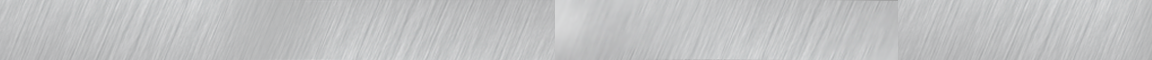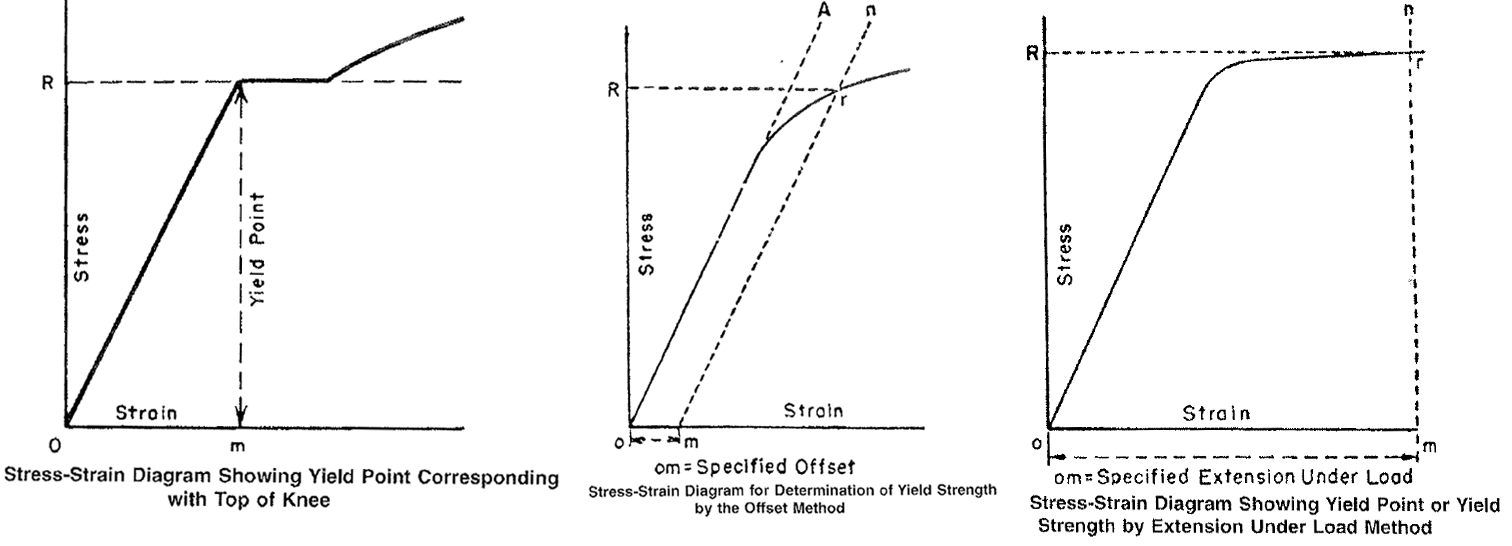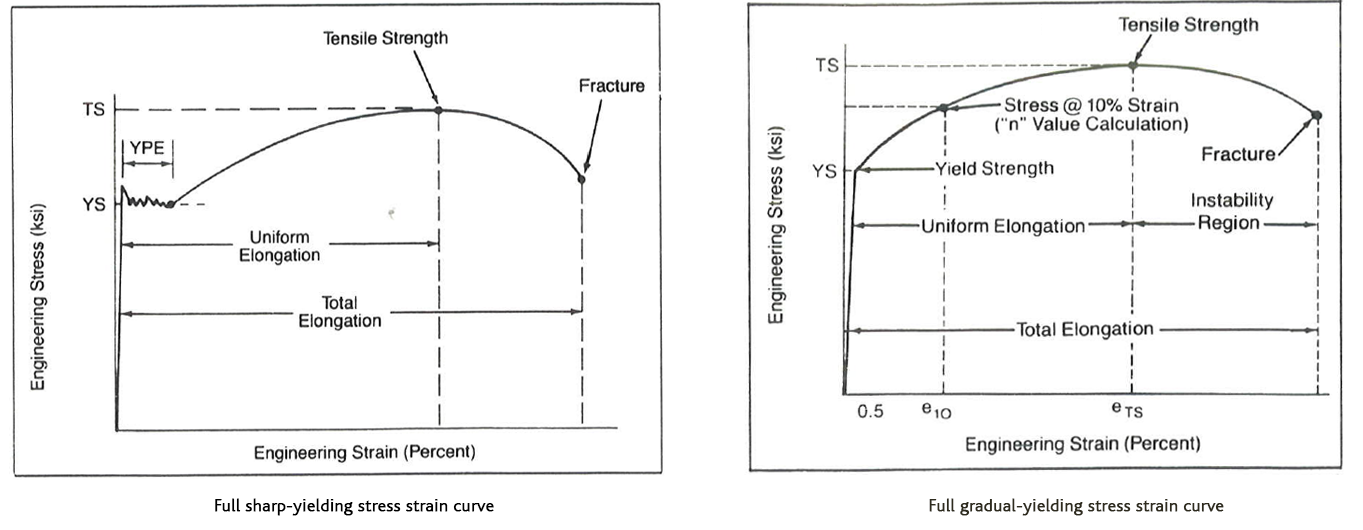# Tensile Testing

by Esther Mar | Sep 28, 2017
• The uni-axial tension test, or tensile test, provides a great deal of information about material characteristics.
• In the case of sheet products a full thickness sample, about 8” long by ¾” wide, is machined to have a 2” long x ½” wide “reduced section”.
• The machined coupon is pulled in tension to breakage while the load and the resulting extension are recorded simultaneously.This is done in a tensile testing machine.• The common graph generated by this test is the Engineering Stress-Strain curve which plots Engineering Stress (load divided by original cross-sectional area) on the Y-axis against Strain (extension in %) on the X-axis.
• Young’s Modulus (Elastic Modulus) – This is the slope of the initial, linear, part of the curve where any increase in load results in a proportional increase in strain. The end of the linear portion of the curve is referred to as the Proportional Limit.
• Yield Point – This is the first stress, less than the maximum obtainable stress, at which an increase in strain occurs without an increase in stress. Such behaviour is only common to certain materials. These materials are said to exhibit a yield point elongation.
• In many materials the behaviour beyond the end of the end of the Proportional limit is smooth.In such materials the Yield Strength is determined through conventions called “% offset” or “extension under load”.• The Tensile Strength (used to be called the Ultimate Tensile Strength) is the stress obtained at the maximum load the specimen sustains during the tension test divided by the original cross section of the coupon.
• Before the Tensile Strength is achieved the material is said to exhibit uniform thinning, i.e. the whole coupon deforms uniformly under increasing load. Up to this point the elongation exhibited is referred to as the uniform elongation.
• After load reaches the maximum (Tensile strength is obtained) the material is said to exhibit non-uniform thinning (demonstrating a reduction in width, then a reduction in thickness called localized necking and eventually fracture.)
• The elongation obtained at fracture is referred to as the total elongation.
• The total strain is the strain up to the Tensile Strength and consists of two portions: Elastic and Plastic.
• Elastic Strain relaxes (totally or partially) resulting in elastic recovery, or spring back.
• Plastic Strain remains, resulting in the final shape of the part produced.
• In order to form a part, stresses must be applied within the Plastic region of the curve, i.e. past the Yield Strength.• The n-value – work hardening exponent is related to the steepness of the stress-strain curve in the plastic deformation region.It also correlates with the uniform elongation of the steel.It correlates to the material’s ability to be stretch formed, i.e. to work harden or to distribute deformation uniformly so the higher the n-value the less localized deformation.# ARCHIVE

Do you an idea, feedback or remark for our blog? Drop us a line in the comment section or email us at info@baileymetalprocessing.com.